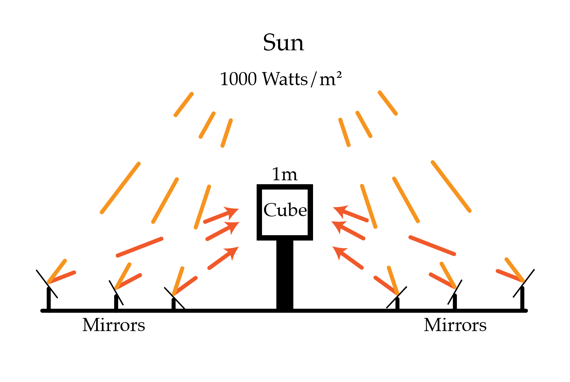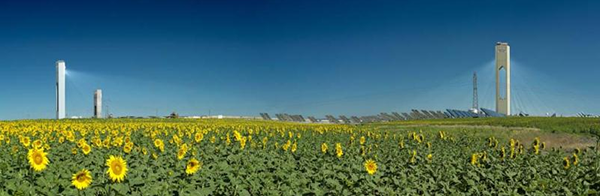# Behold The Power Of The SunSolar collector power generation plants, such as this one in Spain, work by concentrating solar energy into a small region to drive a steam turbine.Direct sunlight delivers about $1000~\mbox{Watts/m}^2$ of energy in the infrared, visible, and ultraviolet parts of the spectrum. I want to use mirrors with a total effective area $A$ to direct this solar energy into a cube $1~\mbox{m}$ on a side, heating the cube to a steady $100^\circ$ Celsius so I can start generating steam and running a turbine. What is the necessary effective mirror surface area $A$ in $\mbox{m}^2$ to do this?

Note that your $A$ will be smaller than you might expect. This is because we have used components that are 100% efficient - all the solar energy gets directed onto the cube. In a real life situation the amount of solar energy redirected by the mirrors is much, much less.

Assumptions

• The temperature of the air is 20 $^\circ$C

Image credit: Abengoa

×

Problem Loading...

Note Loading...

Set Loading...